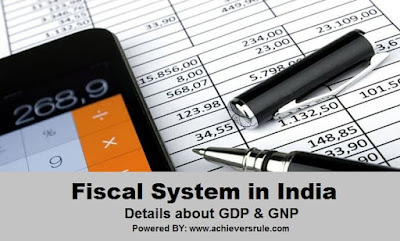# Fiscal System in India

##Fiscal System in India - Details about GDP and GNP

• GNP is the best measure of economic growth of a country.
• Since 1951, in India national income and per capita income has also increased but at a slower rate.
• The Government make arrangement for the collection of revenues for a year by- Finance Bill.
• Export –import method is not a method of estimating national income.
• The national income of India mainly through- Production and income methods.
• The system of Budget was introduced in India during the viceroyalty of – Lord Canning.
• One of the problems in calculating the national income in India correctly is – non monetised consumption.
• A deflator is a technique of- adjusting for changes in price level.
• National income of India is compiled by – Central Statistical Organisation.
• The main cause of slow rate of growth of per capita income in India- is 1). High capital-output ratio and 2). High rate of growth of population.
• Contribution of Household sector is the largest in the Gross Domestic Saving in India.
• Per capita income is not required while computing Gross National Product.
• National income is same as- Net National Product at factor cost.
• Net Factor income from abroad to GDP gives-GNP.
• Gross National income is always more than Net National Income because it includes- capital consumption allowance.

Purchasing, Power, Parity: Assume that 1 dollar equals to Rs.60. This dollar when use in America and when the Rs. 60 use in India , then the result should be very different. Rs. 60 in India will be far more valuable than America. The formula is derived using this logic can be used to calculated GDP & PPP.

GDP calculation:
a). GDP is the market value of all goods and services produced with in a country border, in a given year.
b). GDP per capita=GDP/population.c). This measure the standard of living.
d). GDP can be calculated in three ways, all given same result.
Method 1:
Expenditure Method:
This method works on the principles that, all of the products (goods and services) must be purchased by somebody, hence the value of total product must be equal to people expenditure buying things.
Formula:
GDP=C + I + G + (x-m)

Where:
• C=Pvt. Consumption=all first time purchase goods & services.
• I=Gross investment=Investment in Shares, Banks, Branches etc.
• G=Government Spending=All governmental spending (Salaries, Purchase etc).
• x-m=Export - Import.
Method 2:
Production Method: GDP is the total money value of all goods and services calculated during any one year. We have to cheque that net value added in each stage, across all lines.
Formula:
GDP(mp)=GDP(fc) +Indirect Tax – Subsidy
Where:
• GDP (fc) =money value of everything produce, without counting any Government rule.
• GDP= Sum total of gross value/all farms in economy.
Farmer – Wheat production – sales to mill – 10kg@ Rs. 500.
Mill – ground it – sold to bakery-10kg @ Rs. 600.
Bakery – makes brad – sold to customers – 10 kg @ Rs. 800.
What GDP was purchased -> @ Rs. (500 + 600 + 800) =Rs. 1900 is wrong.
GDP produced will be money value of this line Rs.(500+100+200)= Rs. 800.

Factors cost means Original Value.
Method 3:
Income Method: GDP is the sum of total incomes of individual leavings in a country in a given year.
Revenue earned by all the farms put together must be distributed among the factors of production, as Salaries, wages, profits, interest earning and rents.
Formula:
GDP=W + P + In + R
Where:
• W=Salary + wages.
• P=Profit.
• In=Interest earnings.
• R=Rent.
Extra Notes:
• Fiscal Deficit: Difference between Total Expenditure and Revenue Receipts plus non-debt creating capital receipts.
• Revenue Deficit: Difference between Total Expenditure and total receipts.
• Revenue Deficit: Difference between Revenue Expenditure and Revenue receipts.
• Primary Deficit: Difference between Total Expenditure and revenue Receipts plus non-debt creating capital receipts minus interest payments.